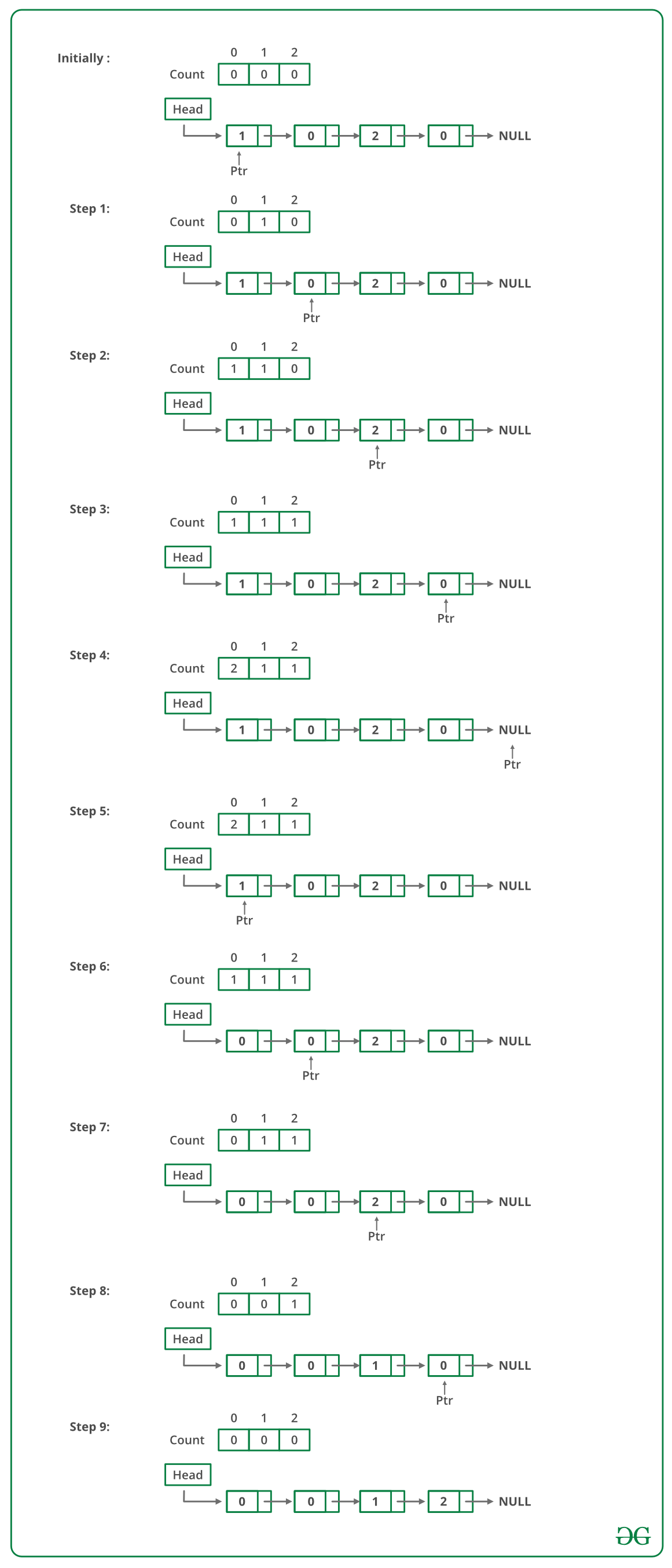# C Program For Sorting A Linked List Of 0s, 1s And 2s

• Last Updated : 22 Dec, 2021

Given a linked list of 0s, 1s and 2s, sort it.
Examples:

```Input: 1 -> 1 -> 2 -> 0 -> 2 -> 0 -> 1 -> NULL
Output: 0 -> 0 -> 1 -> 1 -> 1 -> 2 -> 2 -> NULL

Input: 1 -> 1 -> 2 -> 1 -> 0 -> NULL
Output: 0 -> 1 -> 1 -> 1 -> 2 -> NULL
```

Source: Microsoft Interview | Set 1

Following steps can be used to sort the given linked list.

• Traverse the list and count the number of 0s, 1s, and 2s. Let the counts be n1, n2, and n3 respectively.
• Traverse the list again, fill the first n1 nodes with 0, then n2 nodes with 1, and finally n3 nodes with 2.

Below image is a dry run of the above approach:Below is the implementation of the above approach:

## C

 `// C Program to sort a linked list ``// 0s, 1s or 2s``#include``#include`` ` `// Link list node ``struct` `Node``{``    ``int` `data;``    ``struct` `Node* next;``};`` ` `// Function to sort a linked list ``// of 0s, 1s and 2s``void` `sortList(``struct` `Node *head)``{``    ``// Initialize count of '0', '1' ``    ``// and '2' as 0``    ``int` `count = {0, 0, 0};  ``    ``struct` `Node *ptr = head;`` ` `    ``/* Count total number of '0', '1' and '2'``       ``count will store total number of '0's``       ``count will store total number of '1's``       ``count will store total number of '2's  */``    ``while` `(ptr != NULL)``    ``{``        ``count[ptr->data] += 1;``        ``ptr = ptr->next;``    ``}`` ` `    ``int` `i = 0;``    ``ptr = head;`` ` `    ``/* Let say count = n1, count = n2 and ``       ``count = n3``       ``now start traversing list from head node,``       ``1) fill the list with 0, till n1 > 0``       ``2) fill the list with 1, till n2 > 0``       ``3) fill the list with 2, till n3 > 0  */``    ``while` `(ptr != NULL)``    ``{``        ``if` `(count[i] == 0)``            ``++i;``        ``else``        ``{``            ``ptr->data = i;``            ``--count[i];``            ``ptr = ptr->next;``        ``}``    ``}``}`` ` `// Function to push a node ``void` `push (``struct` `Node** head_ref, ``           ``int` `new_data)``{``    ``// Allocate node ``    ``struct` `Node* new_node =``           ``(``struct` `Node*) ``malloc``(``sizeof``(``struct` `Node));`` ` `    ``// Put in the data  ``    ``new_node->data  = new_data;`` ` `    ``// Link the old list off the new node ``    ``new_node->next = (*head_ref);`` ` `    ``// Move the head to point to the ``    ``// new node ``    ``(*head_ref)    = new_node;``}`` ` `// Function to print linked list ``void` `printList(``struct` `Node *node)``{``    ``while` `(node != NULL)``    ``{``        ``printf``(``"%d  "``, node->data);``        ``node = node->next;``    ``}``    ``printf``(``"n"``);``}`` ` `// Driver code``int` `main(``void``)``{``    ``struct` `Node *head = NULL;``    ``push(&head, 0);``    ``push(&head, 1);``    ``push(&head, 0);``    ``push(&head, 2);``    ``push(&head, 1);``    ``push(&head, 1);``    ``push(&head, 2);``    ``push(&head, 1);``    ``push(&head, 2);`` ` `    ``printf``(``    ``"Linked List Before Sorting"``);``    ``printList(head);`` ` `    ``sortList(head);`` ` `    ``printf``(``    ``"Linked List After Sorting"``);``    ``printList(head);`` ` `    ``return` `0;``}`

Output:

```Linked List Before Sorting
2  1  2  1  1  2  0  1  0